# Coupled one-storey plane framesIntroduction >
The structural frame
The construction
The reinforcement I
The reinforcement II
Quantity/Cost estimation
Detailing drawingsIntroduction >
Wind and Seismic Forces >
Structural model and Analysis
Modelling slabs
Slabs
Seismic behavour of frames
Appendix A
Appendix B
Appendix C
Appendix DIntroduction >
Materials
To be continued >

## Coupled one-storey plane frames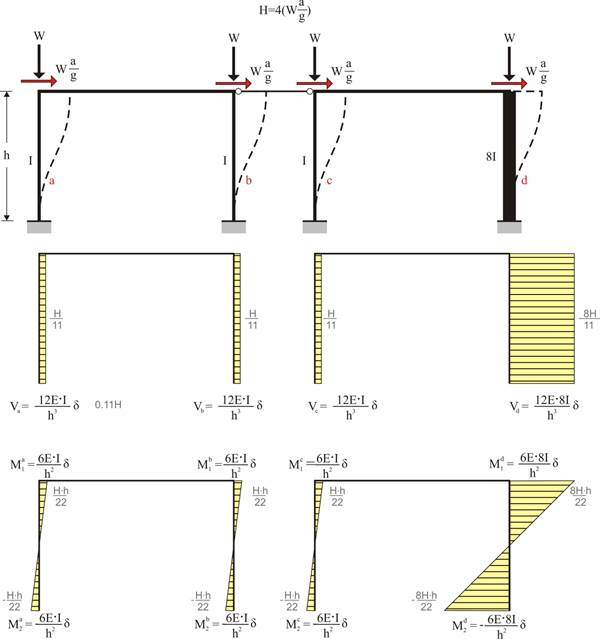As coupled plane frames are defined the plane frames that are connected in such a way that, subject to horizontal forces, they present uniform displacements.

The crossbars of the one-bay frames illustrated in the figure have a practically infinite moment of inertia. Both columns of the first frame have moment of inertia Ι whereas the two columns of the second have Ι and 8Ι respectively.

## The structural frame model fully stiffened, project <B_520>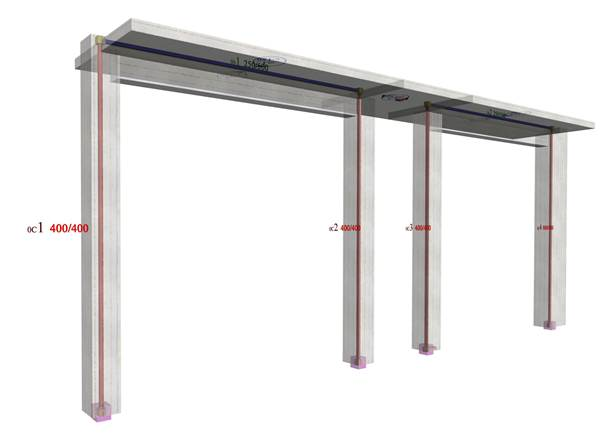In project <B_520> of the related software, the cross-section of columns C1, C2 and C3 is 400/400 and C4 is 800/400. The height of all columns is 5.0 m. The cross-section of the flanged beam is 250/500/1010/150 and its span is 5.0 m.

## Analysis results of project <B_520> with actual beam and column stiffness (graphical representation in the related software)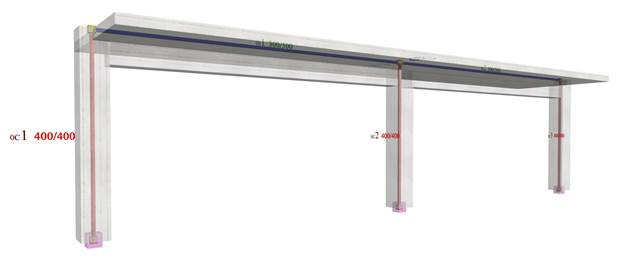Elastic line, δ=8.078 mm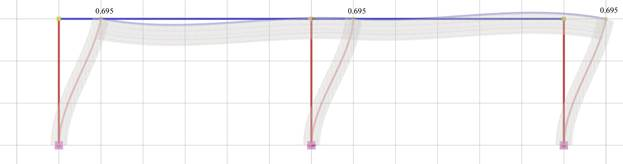Shear force diagrams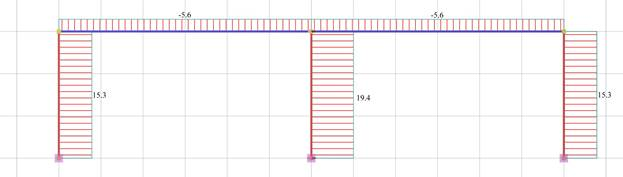Bending moment diagram